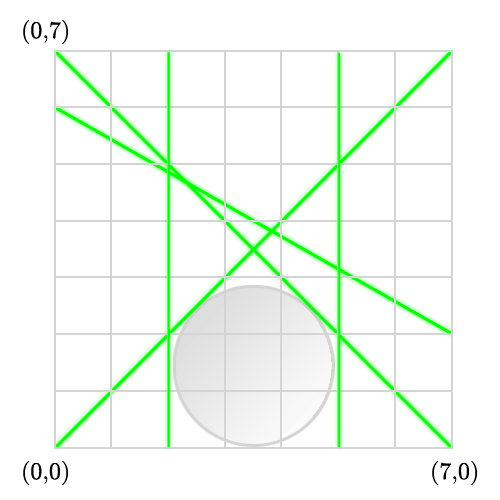## Secret Room

Hmm, now why would someone break into a mundane software company?
After looking at the building blueprints (to place cameras), you've noticed the suspicious room at the top.
Investigating a little, you find no visible entrance - but there's a strange humming noise emanating from the room.

Upon asking your manager you are ushered into the conference room where a confidential meeting seems to be taking place.
It seems that this secret room holds a certain treasure: the thishasbeencensored
Now, they want to secure this room with lethal green laser beams.
The lasers are very thin, but they have the ability to burn a hole through an intruder instantly.

The company engineers have built several laser designs, and they'd like to choose the best one.
To make a laser design effective, they want to minimize the freedom a thief would have while in the room.
As an estimate, they'd like to find the largest circle that could fit anywhere without hitting a laser.
Now, doing this by hand would be quite tedious - so write a program to do it for them!

### Input

Two positive integers, W and H (W,H ≤ 10,000) representing the width and height of the room.
Then, an integer N ≤ 50, representing the number of laser beams.
For each laser beam:
4 integers, (x1,y1),(x2,y2) representing one laser beam.
A laser beam will travel between those two points (they are guaranteed to be on the wall).
The room will be modeled as a 2-D grid - (0,0) being the lower left and (W,H) the upper right.

### Output

The radius of the largest circle that could fit without being cut by lasers.

```7 7
5
0 0 7 7
0 7 7 0
0 6 7 2
2 0 2 7
5 0 5 7```

### Sample Output

`1.4497`

The exact answer is 3.5(√2 - 1) = 1.449747...
(Try calculating it! Please don't try coordinate geometry :P)

### DiagramPoint Value: 25 (partial)
Time Limit: 2.00s
Memory Limit: 16M
Author: hansonw1

Languages Allowed:
C++03, PAS, C, HASK, ASM, RUBY, PYTH2, JAVA, PHP, SCM, CAML, PERL, C#, C++11, PYTH3

• (0/1)
Actually it was a bug - when I resubmitted test data the old submission didn't get updated properly.

• (1/1)
Parallel lines are from HELL.

• (1/1)
That's why you use the PUGA (Plachta Universal Geometry Algorithm) for all geometry problems.

• (1/1)
If it helps, you can use this python program to draw test cases. (I used this to check my test data)
(It is pretty picky with how you input your data - use the same format as the sample input)

``import Tkinter, os from Tkinter import *  W, H = map(int, raw_input("Width and height? (W H) ").split(" "))  wscale = 1. * W / max(W, H) * 800 hscale = 1. * H / max(W, H) * 800  def pt(x, y): 	x = 5 + 1. * x / W * wscale 	y = 5 + hscale - 1. * y / H * hscale 	return x,y  root = Tk()  canvas = Canvas(root, width=wscale+10, height=hscale+10) canvas.pack()  canvas.create_line(pt(0,0), pt(0,H)) canvas.create_line(pt(0,H), pt(W,H)) canvas.create_line(pt(W,H), pt(W,0)) canvas.create_line(pt(W,0), pt(0,0))  N = int(raw_input("Number of lines? (N) ")) for i in range(N): 	x1,y1,x2,y2 = map(int, raw_input("Line: (x1 y1 x2 y2) ").split(" ")) 	canvas.create_line(pt(x1,y1), pt(x2,y2), fill="red")  #If you want, you can draw a circle to see if it lines up	 sz = float(raw_input("Radius of the circle? (R) "))  x, y = map(float, raw_input("Centre of the circle (x y)? ").split(" ")) canvas.create_oval(pt(x-sz,y-sz), pt(x+sz,y+sz))  root.mainloop()``